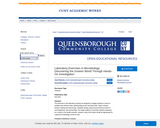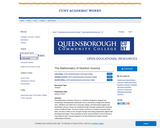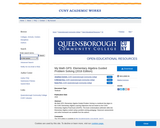Updating search results...

# 3 Results

View
Selected filters:
• Queensborough Community CollegeConditional Remix & Share Permitted
CC BY-NC-SA
Rating
0.0 stars

The exercises in this laboratory manual are designed to engage students in hand-on activities that reinforce their understanding of the microbial world. Topics covered include: staining and microscopy, metabolic testing, physical and chemical control of microorganisms, and immunology. The target audience is primarily students preparing for a career in the health sciences, however many of the topics would be appropriate for a general microbiology course as well.

Lab 1. Introduction to Microscopy and Diversity of Cell Types
Lab 2. Introduction to Aseptic Techniques and Growth Media
Lab 3. Preparation of Bacterial Smears and Introduction to Staining
Lab 4. Acid fast and Endospore Staining
Lab 5. Metabolic Activities of Bacteria
Lab 6. Dichotomous Keys
Lab 7. The Effect of Physical Factors on Microbial Growth
Lab 8. Chemical Control of Microbial Growth—Disinfectants and Antibiotics
Lab 9. The Microbiology of Milk and Food
Lab 10. The Eukaryotes
Lab 11. Clinical Microbiology I; Anaerobic pathogens; Vectors of Infectious Disease
Lab 12. Clinical Microbiology II—Immunology and the Biolog System
Lab 13. Putting it all Together: Case Studies in Microbiology
Appendix I. Information About Lab Practical Exams
Appendix II. Scientific Notation and Serial Dilution
Appendix III. Introduction to Micropipetting

Subject:
Natural Science
Material Type:
Activity/Lab
Provider:
Provider Set:
Queensborough Community College
Author:
Joan Petersen
Susan McLaughlin
04/24/2019Conditional Remix & Share Permitted
CC BY-NC-SA
Rating
0.0 stars

The Mathematics of Nutrition Science is a workbook designed to integrate and contextualize developmental mathematics into an introductory college level Nutrition class. Definitions and skills from Community College Level Elementary Algebra and Quantitative Literacy courses are explained through examples analyzing the nutritional content of different foods. The book contains exercises for students to practice these skills, and also to reflect on the concepts through short writing assignments aligned with developmental English. These materials could be used by Nutrition course instructor in many different ways, and are designed to be self-contained and require minimal mathematical instruction.

Subject:
Natural Science
Nutrition
Material Type:
Textbook
Provider:
Provider Set:
Queensborough Community College
Author:
Jennifer Maloy
Jonathan Cornick
Lana Zinger
03/03/2017Conditional Remix & Share Permitted
CC BY-NC-SA
Rating
0.0 stars

My Math GPS: Elementary Algebra Guided Problem Solving is a textbook that aligns to the CUNY Elementary Algebra Learning Objectives that are tested on the CUNY Elementary Algebra Final Exam (CEAFE). This book contextualizes arithmetic skills into Elementary Algebra content using a problem-solving pedagogy. Classroom assessments and online homework are available from the authors.

Linear Equations And Inequalities

GPS 1: Understanding Linear Equations
GPS 2: Solving Linear Equations With Whole Numbers
GPS 4: Subtracting Signed Numbers
GPS 5: Multiplying And Dividing Signed Numbers
GPS 6: Solving Linear Equations With Signed Numbers
GPS 7: Translating Words Into Expressions And Equations
GPS 8: Solving Linear Inequalities, Part 1
GPS 9: Solving Linear Inequalities, Part 2
GPS 10: Solving Linear Equations With Fractions
GPS 11: More Linear Equations With Fractions
GPS 12: Solving Literal Linear Equations
The Coordinate Plane And Lines

GPS 13: Introduction To The Coordinate Plane
GPS 14: Intercepts Of A Line
GPS 15: Slope And Equations Of A Line
GPS 16: The Slope-Intercept Equation Of A Line
GPS 17: Slope-Intercept Equation And Graphing
GPS 18: Graphing Lines In Slope-Intercept Form
GPS 19: Finding An Equation Of A Line From Its Graph
GPS 20: Horizontal And Vertical Lines
Systems Of Linear Equations

GPS 21: Introduction To Systems Of Linear Equations And Solving Graphically
GPS 22: More On Solving Systems Graphically
GPS 23: Solving Systems Of Linear Equations Algebraically
GPS 24: More On Solving Systems Algebraically
Exponents

GPS 25: Rules Of Exponents, Part 1
GPS 26: Rules Of Exponents, Part 2
Polynomials And Operations

GPS 27: Introduction To Polynomials And Operations
GPS 28: Multiplying Polynomials
GPS 29: Removing The Greatest Common Factor
GPS 30: Factoring By Grouping
GPS 31: Factoring Trinomials By Grouping – Part 1
GPS 32: Factoring Trinomials By Grouping – Part 2
GPS 33: Factoring Trinomials By Grouping – Part 3
GPS 34: Factoring Trinomials By Grouping – Part 4
GPS 35: Factoring A Difference Of Squares
GPS 36: Multistep Factoring
GPS 37: Solving Quadratic Equations By Factoring
Algebraic Expressions

GPS 38: Evaluating Algebraic Expressions
Square Roots And Operations

GPS 39: Introduction To Square Roots
GPS 40: Operations With Square Roots
GPS 41: Pythagorean Theorem

Subject:
Algebra
Mathematics
Material Type:
Textbook
Provider: